>旅游>正文

# 来越南之前最好知道这些！

————欢迎关注微信公众账号越南印象————

——北京时间8am时，越南是7am

^^^^^^^^^^^^^^^^^^^^^^^^^^^^^^

1、旅游签1个月一次往返

2、商务签3个月多次往返（需邀请函）

3、越南驻华使馆信息

^^^^^^^^^^^^^^^^^^^^^^^^^^^^^^

46 Hoang Dieu, Ba Dinh, Hanoi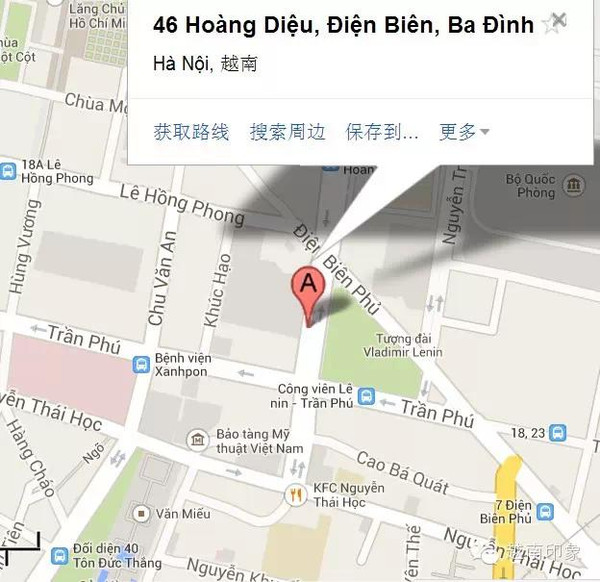No.39，Nguyen Thi Minh Khai Street, Ho Chi Minh city

^^^^^^^^^^^^^^^^^^^^^^^^^^^^^^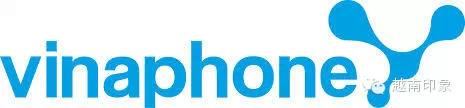3G包流量套餐（2014年3月）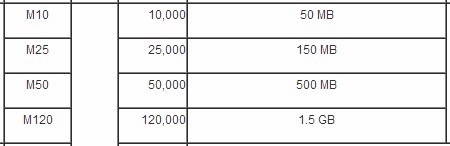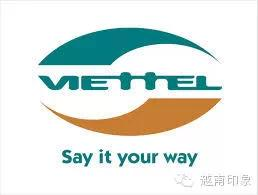3G包流量套餐（2014年3月）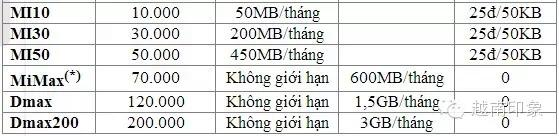^^^^^^^^^^^^^^^^^^^^^^^^^^^^^^

1、越航（服务好、价格略高）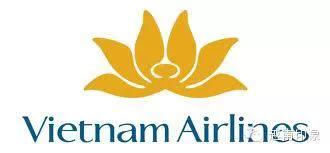2、Jetstar（国际知名廉价航空）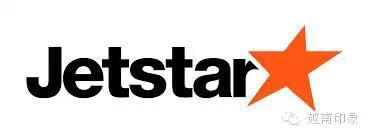3、Vietjet（本地廉价航空）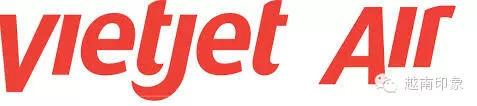^^^^^^^^^^^^^^^^^^^^^^^^^^^^^^

1）在目的地自助出游，可考虑租摩托车

150千越南盾租24小时

2）出租车：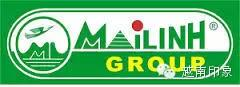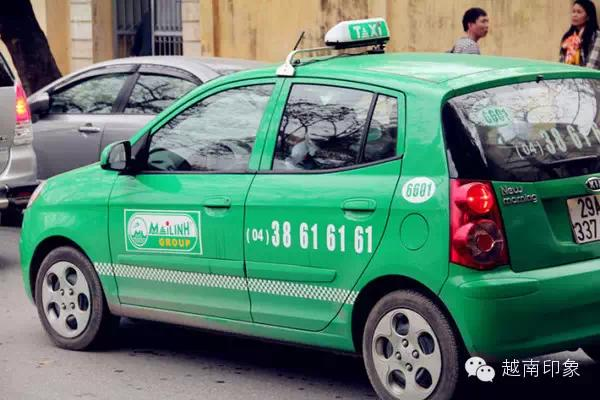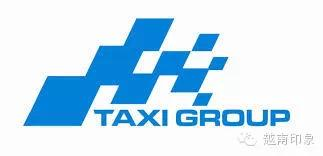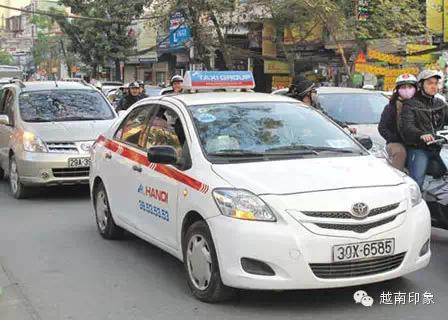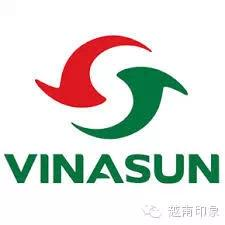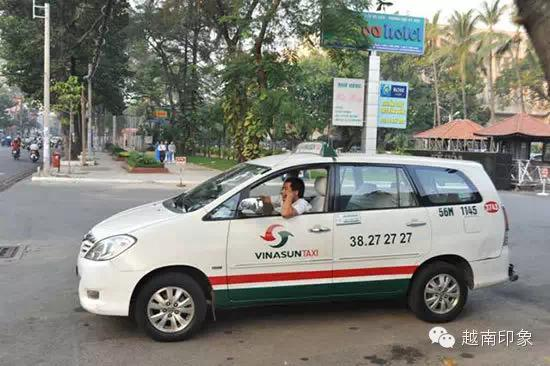^^^^^^^^^^^^^^^^^^^^^^^^^^^^^^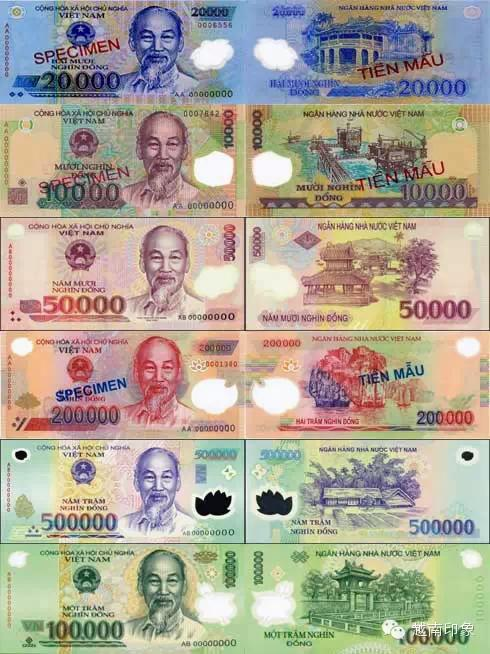^^^^^^^^^^^^^^^^^^^^^^^^^^^^^^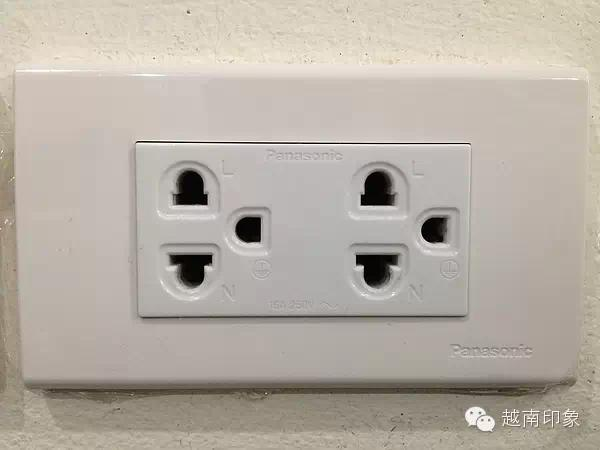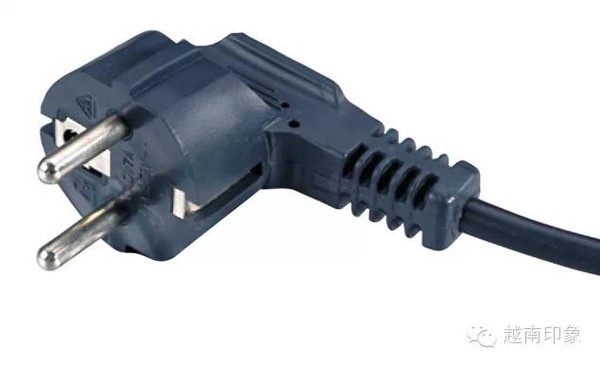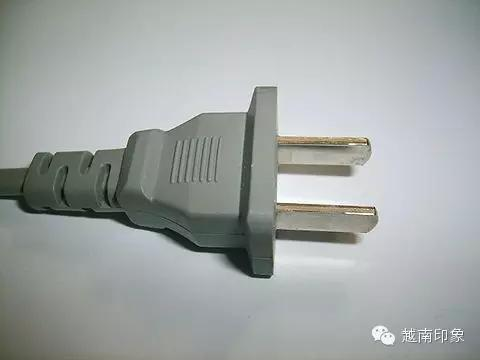^^^^^^^^^^^^^^^^^^^^^^^^^^^^^^

1）36 Phố Phương Liệt——还剑湖夜市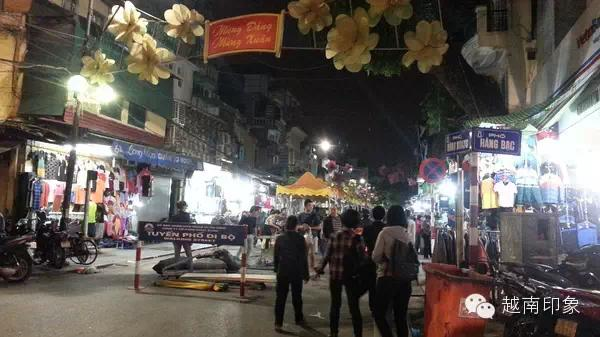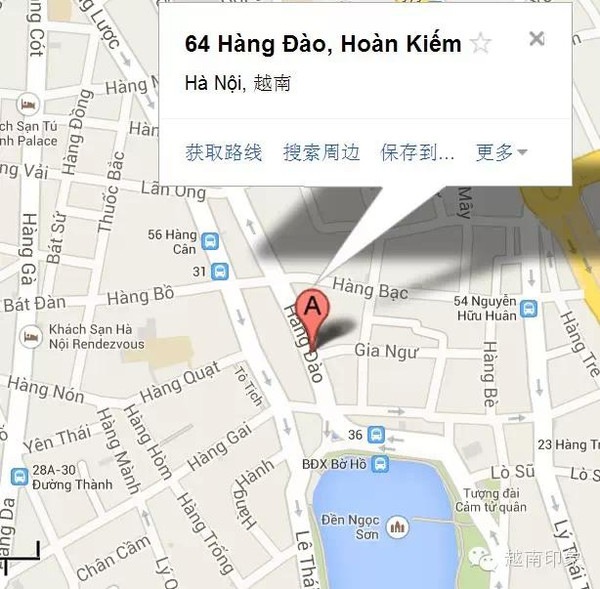2）大型商场、超市——Royal City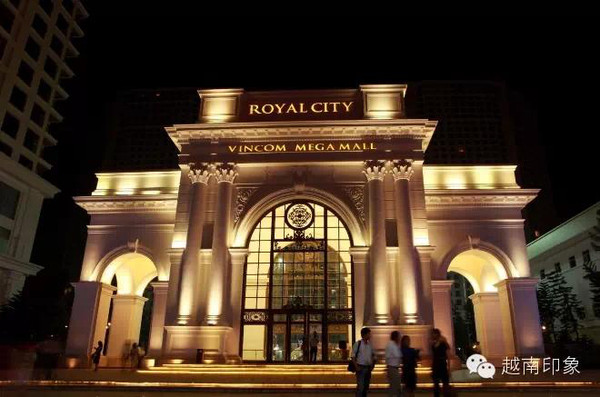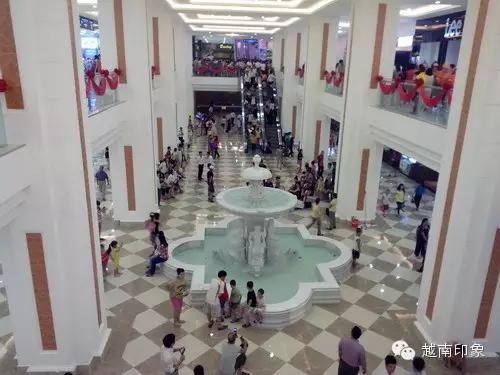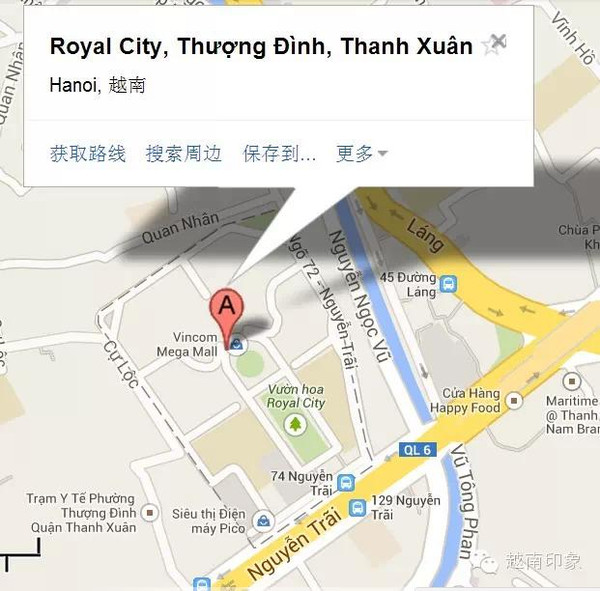^^^^^^^^^^^^^^^^^^^^^^^^^^^^^^